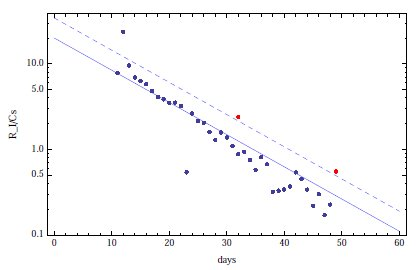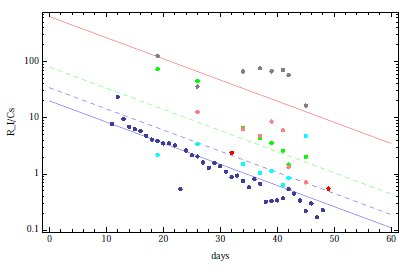### Ratios of Iodine-131 to Cesium-137 at the Fukushima reactors

A few days ago it was uploaded on arXiv a preprint about radiactions from Fukushima reactors, Deciphering the measured ratios of Iodine-131 to Cesium-137 at the Fukushima reactors, by T. Matsui (University of Tokyo).
In the preprint, Matsui propose some simple theoretical calculations to evaluate the situation of TEPCO's reactors.
The physical basis is the radioactive decay,
$N(t) = N_0 e^{-\lambda t}$
where $N_0$ is the number of nucleus at time $t_0$ (in the beginning), $\lambda$ is the decay rate (inverse of life time $\tau$), $N (t)$ is the number of nucleus at time $t$.
To calculate the equation we start from the experimental law,
$\frac{\text d N (t)}{\text d t} = - N(t) \lambda$
For his calculation, Matsui used the following differential equation
$\frac{\text d N_I (t)}{\text d t} = f_I N_0 \theta (t;t_i, t_f) - \lambda_I N_I$
where $\theta (t;t_i, t_f) = 1$ for $t_i < t < t_f$ and $\theta (t;t_i, t_f) = 0$ otherwise, $N_0$ is the number of fission in time, $f_I$ is the fraction of I-131 produced in every fission, $\lambda_I$ the decay rate of I-131. Boundary conditions are: nuclear reactor had been in operation from $t_i$ to $t_f$; $N_I (t_i) = 0$. After integration, and introducing the condition $\lambda_I (t_f - t_i) \gg 1$ (it means that the working time of the reactor is much longer than the half-life of I-131, that is 8 days), Matsui found:
$N_I (t) \simeq \frac{f_I N_0}{\lambda_I} e^{-\lambda_I (t-t_f)}$
Similar calculation for Cs-137:
$N_{Cs} (t) \simeq f_{Cs} N_0 \Delta t e^{\lambda_{Cs} (t-t_f)}$
where $\Delta t = t_f - t_i$ and, because $\tau_{Cs}$ is 30 years, there is the following approximation: $\lambda_{Cs} (t_f - t_i) \ll 1$ (it means that the reactor works for a time much less than the half-life of Cs-137).
So we can calculate decay rates
$\Gamma_X = -\frac{\text d N_X (t)}{\text d t}$
and the ratio:
$R (t) = \frac{f_I}{f_{Cs}} \frac{\tau_{Cs}}{\Delta t \ln 2} \left ( \frac{1}{2} \right )^{(t-t_f)/\tau_I}$
Finally Matsui confront theoretical calculation with data released by TEPCO. He produced the following two plots:Ratio of the radioactivities of I-131 and Cs-137 plotted against number of days since the earthquake (March 11, 2011). The blue solid line ($\Delta t$ = 12 months) and the blue dashed line ($\Delta t$ = 7 months) are the theoretical lines based on the ratio formula; the blue dots are the measured ratios from the samples of sea water taken at the southern monitoring post. The red dots are the data of the water samples of the unit-4 reactor cooling pool.

For the second plot, Matsui calculates a ratio-bis formula:
$R^{\text{new}} (t) = \frac{f_I}{f_{Cs}} \frac{\tau_{Cs}}{\tau_I} e^{(t-t'_f)}$Same plot as fig. 1, now including the data of the water samples taken from the sub-drains near the unit 1 (green), unit 2 (gray), unit 3 (cyan) and unit 4 (pink) reactor buildings. Two more theoretical lines are shown as a guide to the eye; they are computed from ratio formula with $\Delta t$ = 3 months (dashed green) and ratio-bis formula with $t′_f = t_X$ (solid red) which gives an upper bound if the fission ends on X-day.

Repleacing $t'_f$ with $t_X$, that is the time of earthquake, produces an upper bound on the radioactivity ratio.
From the first plot we can conclude that data is better fit using $\Delta t$ = 1 year, but from second plot, unit-2 and unit-4 present important anomaly.
Regarding unit-4:
If the data are correct, it would imply that at least some of the radioactive fission products found in the unit-4 cooling pool have been produced by nuclear reactions that took place at time $t_X$ or later. One possible explanation could be contamination by fallout of the fission products created in the neighboring reactors. Another possible explanation could be that a nuclear chain reaction was reignited in the melted used fuel in the unit-4 cooling pool for a certain period.
And for unit-2:
If there is no strong chemical filtering effect in draining contaminated water from the reactor buildings, it would be difficult to understand the observed anomaly near the unit-2 reactor without assuming that a significant amount of fission products were produced at least 10 - 15 days after X-day.
In conclusion:
some of these fission products were produced by chain nuclear reactions reignited after the earthquake.
It's very important to remember the hypotesis of the work:
• Following Libby, Anderson and Arnold, Matsui's ratio should decrease on the time scale of days after the termination of nuclear fission processes and hence may be used for measuring the age of the fission products, similar to the carbon dating method using the ratio of C-14 to C-12 in the remains of ancient life. But there are two problems: the measured ratio depend by the controlled nuclear reaction; the effects of the different chemical properties of iodine and cesium.
• Matsui assumed that the solubility of iodine or cesium in water is not changed considerably by boric acid, which has been added to the water to suppress chain nuclear
reactions.
• The total amount of the radioactivity by I-131 contained in the unit 4 cooling pool, which normally contains about 1500 tons of water, may be estimated as 220×1.5×109 = 3.3×1011 Bq which is small compared to the radioactivity of fresh fission products contained in the nuclear reactor, which is the order of 1018 Bq.
In every case, the paper seems indicate a very difficult situation around reactor.

(via Nature blog)